# Algebra - math word problems

1. Reciprocal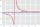It is true (prove it) that if a> b> 0: ?
2. Arc and segmentCalculate the length of circular arc l, area of the circular arc S1 and area of circular segment S2. Radius of the circle is 11 and corresponding angle is ?.
3. RootsDetermine the quadratic equation absolute coefficient q, that the equation has a real double root and the root x calculate: ?
4. Diofant 2Is equation ? solvable on the set of integers Z?
5. Pumps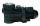The tank is filled with two pumps in 16 minutes. The first pump is filled in 30 minutes earlier than two one. How many minutes is filled with the first pump?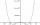By introducing a new variable solve biquadratic equation: ?
7. Determine the number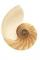Determine the number x that ?.
8. Subsets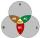How many are all subsets of set ??Determine the numbers b, c that the numbers x1 = -1 and x2 = 3 were roots of quadratic equation: ?
10. Diofant equationIn the set of integers (Z) solve the equation: ? Write result with integer parameter ? (parameter t = ...-2,-1,0,1,2,3... if equation has infinitely many solutions)
11. 3-bracket 2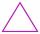May be the smallest angle in the triangle greater than 40°?
12. 3-bracketMay be the largest angle in the triangle less than 20°?
13. 3-bracket 3Two angles in a triangle are 90° and 60°. Has triangle at least two equal sides?
14. Rectangular fieldOne dimension of the rectangular field is 56 m greater than second dimension. If each side of the rectangle increases by 10 m, increases the surface field is 1480 m2 . Determine dimencions of the field.
15. Euler problem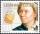Someone buys a 180 tolars towels. If it was for the same money of 3 more towels, it would be 3 tolars cheaper each. How many were towels?
16. Number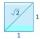Is number 5.146852 irrational?
17. Special cube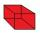Calculate the edge of cube, if its surface and its volume is numerically equal number.
18. Rates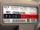When gas consumption, the consumer may choose one of two rates: rate A - which pays 0.4 € per 1 m3 of gas a flat monthly fee of 3.9 € (regardless of consumption) rate B - which pays 0.359 € per 1 m3 of gas a flat monthly fee of 12.5 € From what month
19. Exponential equationIn the set R solve the equation: ?
20. Proof I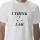When added to the product of two consecutive integers larger one, we get square larger one. Is this true or not?

Do you have an interesting mathematical word problem that you can't solve it? Submit math problem, and we can try to solve it.

We will send a solution to your e-mail address. Solved examples are also published here. Please enter the e-mail correctly and check whether you don't have a full mailbox.

Please do not submit problems from current active competitions such as Mathematical Olympiad, correspondence seminars etc...